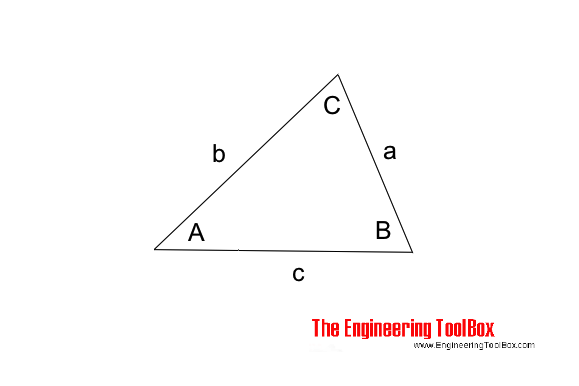Engineering ToolBox - Resources, Tools and Basic Information for Engineering and Design of Technical Applications!

# Law of Tangents

## Triangles and law of tangents.

The "Law of Tangents" can be used to calculate the angles or sides of a triangle.### Law of Tangents

The "Law of Tangents" can be expressed as

(a + b) / (a - b) = tan 1/2 (A + B) / tan 1/2 (A - B)                         (1)

where

a, b and c = length of sides in triangle (m, ft ...)

A, B and C = angles in the triangle (degrees)

### Pythagorean theorem

The Pythagorean theorem can be expressed as

a2 + b2 = c2                 (2)

### Law of sines

The Law of sines can be expressed as

a / sin(A) = b / sin(B) = c / sin(C)                (3)

### Angles

If the length of all three sides in the triangle are known - the angles can be calculated as

A = arccos((b2 + c2 - a2) / (2 b c))                     (4a)

B = arccos((a2 + c2 - b2) / (2 a c))                     (4b)

C = arccos((a2 + b2 - c2) / (2 a b))                     (4c)

## Related Topics

• Mathematics - Mathematical rules and laws - numbers, areas, volumes, exponents, trigonometric functions and more.

## Engineering ToolBox - SketchUp Extension - Online 3D modeling!

Add standard and customized parametric components - like flange beams, lumbers, piping, stairs and more - to your Sketchup model with the Engineering ToolBox - SketchUp Extension - enabled for use with the amazing, fun and free SketchUp Make and SketchUp Pro .Add the Engineering ToolBox extension to your SketchUp from the SketchUp Pro Sketchup Extension Warehouse!

Translate

## Privacy

We don't collect information from our users. Only emails and answers are saved in our archive. Cookies are only used in the browser to improve user experience.

Some of our calculators and applications let you save application data to your local computer. These applications will - due to browser restrictions - send data between your browser and our server. We don't save this data.

## Citation

• Engineering ToolBox, (2020). Law of Tangents. [online] Available at: https://www.engineeringtoolbox.com/law-tangents-d_2178.html [Accessed Day Mo. Year].

Modify access date.

. .

#### Scientific Online Calculator6 24